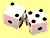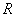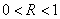## Monte Carlo Simulation

Monte Carlo Simulation is one of the largest and most important classes of numerical method for computer simulations or computer experiments. Monte Carlo simulation can be defined as a method to generate random sample data based on some known distribution for numerical experiments. In shortsighted view, Monte Carlo Simulation always involves random number (though actually not all methods that involve random number can be categorized as Monte Carlo Simulation, they may be better described as part of Monte Carlo Method). Generation of pseudo random numberthat distributed uniformly over intervalis the heart of any Monte Carlo simulation. The random number generated must be independent (no correlation to other random number)

### What is Simulation?

Simulation is emulation of reality using mathematical model

### Why do we need simulation?

A simulation model allow to examine system behavior under different scenarios in virtual computational world. It can be used to identify bottlenecks in a process, provide a safe, and relatively very cheap (in term of both cost and time) testbed to evaluate the side effects and to optimze the performance of the system before transferring them to real world.

### Do not use simulation if ...

• Simulation requires months or years of CPU time. It is surely not worth to try.
• The analytical solution exists and simple. It is easier to use the analytical solution to solve your problem rather than using simulation (unless you want to compare the analytical solution with simulation).

Use simulation when the analytical solution either does not exist, or too complicated or require more computation time than the simulation.

### What are the characteristics of Monte Carlo Simulation?

I would like to emphasis in three characteristics of Monte Carlo Simulation:

1. Random sample generation
2. The input distribution is known
3. Numerical Experiments

The direct output of Monte Carlo simulation method is generation of Random sampling. Other performance or statistical outputs are indirect method, which depend on the applications.

The input of the simulation is distribution (based on some theoretical distribution or from observation)

Numerical experiments characteristic of Monte Carlo simulation lead us to run many samples before we can get the result. Only single sample of the simulation will surely give false results. This happens because Monte Carlo Simulation work based on Monte Carlo Algorithm (see below)

### How the Monte Carlo Algorithm works?

Monte Carlo Algorithm works based on the Law of Large Numbers . It says that if you generate large number of samples, eventually you will get the approximate desire distribution.

Preferable reference for this tutorial is

Teknomo, Kardi. Monte Carlo Simulation Tutorial. https:\\people.revoledu.com\kardi\ tutorial\Simulation